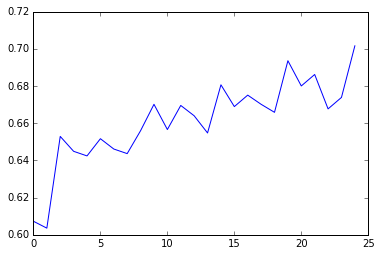# ML Assignment 2: Do teens have sex in Random Forests?

Previously I had examined what “risky attitudes” were associated with teens having had sex using a classification tree. However, the output was impossible to read, so I had to pare down the number of variables to something more manageable… 3. I learned, it seemed, that guilt was a strong motivating factor in preventing teens from having sex. This week we learned about random forests, which essentially involves creating a bunch of classification trees in order to find out which variables are meaningful (would’ve been useful prior to last weeks paring down…). The full Jupyter notebook from which this post was derived can be found here

### Imports

``````%matplotlib inline
from pandas import Series, DataFrame
import pandas as pd
import numpy as np
import os
import matplotlib.pylab as plt
from sklearn.cross_validation import train_test_split
from sklearn.tree import DecisionTreeClassifier
from sklearn.metrics import classification_report
import sklearn.metrics
# Feature Importance
from sklearn import datasets
from sklearn.ensemble import ExtraTreesClassifier
``````

``````columns = ["H1CO1","H1MO1","H1MO2","H1MO3","H1MO4","H1MO5","H1MO8","H1MO9","H1MO10","H1MO11","H1MO13","H1RE4","H1RE6","H1BC8","H1BC7","BIO_SEX"]

data_clean = data_filter1[~data_filter1['H1MO4'].isin([6,7,8,9])]

data_clean.dtypes
``````

## Modeling and Prediction

### Split into training and testing sets

``````predictors = data_clean[["H1MO1","H1MO2","H1MO3","H1MO4","H1MO5","H1MO8","H1MO9","H1MO10","H1MO11","H1MO13","H1RE4","H1RE6","H1BC8","H1BC7","BIO_SEX"]]

targets = data_clean.H1CO1

pred_train, pred_test, tar_train, tar_test  =   train_test_split(predictors, targets, test_size=.4)

``````

### Build model on training data

``````from sklearn.ensemble import RandomForestClassifier

classifier=RandomForestClassifier(n_estimators=25)
classifier=classifier.fit(pred_train,tar_train)

predictions=classifier.predict(pred_test)

sklearn.metrics.confusion_matrix(tar_test,predictions)
sklearn.metrics.accuracy_score(tar_test, predictions)

# fit an Extra Trees model to the data
model = ExtraTreesClassifier()
model.fit(pred_train,tar_train)
# display the relative importance of each attribute
importances = model.feature_importances_
var_imp = pd.DataFrame(data = {'predictors':list(predictors.columns.values),'importances':importances})
print(var_imp.sort_values(by='importances', ascending=False))
``````

|Importances |Predictors| |———————–| |0.100871 | H1MO3 |0.081053 | H1MO4 |0.075720 | H1MO10 |0.073326 | H1MO5 |0.071211 | H1MO2 |0.070827 | H1BC8 |0.065039 | H1MO11 |0.064851 | H1MO8 |0.062072 | H1MO1 |0.061757 | H1MO9 |0.060818 | H1BC7 |0.060162 | H1RE6 |0.059713 | H1MO13 |0.054818 | H1RE4 |0.037762 | BIO_SEX

It seems I was correct in the variables I thought were important in last week’s assignment since the top three were the same variables. In descending order of relative importance the forest found: sexual guilt, mother getting upset if they had had sex, the embarrassment of a pregnancy resulting from sex, and then, a new variable, “If you used birth control, your friends might think that you were looking for sex.” Gender had the least importance of any variable.

## Investigating Accuracy of Random Forest by Number of Trees

``````trees=range(25)
accuracy=np.zeros(25)

for idx in range(len(trees)):
classifier=RandomForestClassifier(n_estimators=idx + 1)
classifier=classifier.fit(pred_train,tar_train)
predictions=classifier.predict(pred_test)
accuracy[idx]=sklearn.metrics.accuracy_score(tar_test, predictions)

plt.cla()
plt.plot(trees, accuracy)
``````The graph above shows a steadily increasing accuracy with the number of trees run, which implies that multiple decision trees are necessary in order to properly classify the data.

python, machinelearning